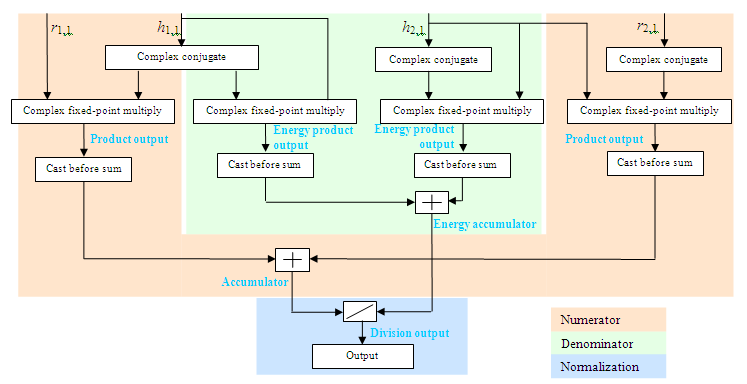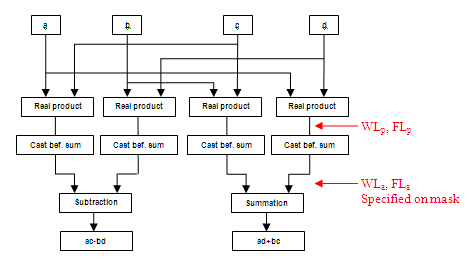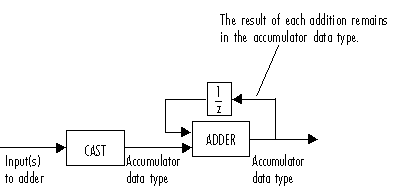# OSTBC Combiner

Combine inputs for received signals and channel estimate according to orthogonal space-time block code (OSTBC)

## Library

MIMO

•## Description

The OSTBC Combiner block combines the input signal (from all of the receive antennas) and the channel estimate signal to extract the soft information of the symbols that were encoded using an OSTBC. The input channel estimate may not be constant during each codeword block transmission and the combining algorithm uses only the estimate for the first symbol period per codeword block. A symbol demodulator or decoder would follow the Combiner block in a MIMO communications system.

The block conducts the combining operation for each symbol independently. The combining algorithm depends on the structure of the OSTBC. For more information, see the OSTBC Combining Algorithms section of this help page.

### Dimension

Along with the time and spatial domains for OSTBC transmission, the block supports an optional dimension, over which the combining calculation is independent. This dimension can be thought of as the frequency domain for OFDM-based applications. The following illustration indicates the supported dimensions for inputs and output of the OSTBC Combiner block.The following table describes each variable for the block.

VariableDescription
FThe additional dimension; typically the frequency dimension. The combining calculation is independent of this dimension.
NNumber of transmit antennas.
TOutput symbol sequence length in time domain.
RSymbol rate of the code.

Note

On the two inputs, T/R is the symbol sequence length in the time domain.

F can be any positive integers. M can be 1 through 8, indicated by the Number of receive antennas parameter. N can be 2, 3 or 4, indicated by the Number of transmit antennas parameter. The time domain length T/R must be a multiple of the codeword block length (2 for Alamouti; 4 for all other OSTBC). For N = 2, T/R must be a multiple of 2. When N > 2, T/R must be a multiple of 4. R defaults to 1 for 2 antennas. R can be either $\frac{3}{4}$ or $\frac{1}{2}$ for more than 2 antennas.

The supported dimensions for the block depend upon the values of F and M. For one receive antenna (M = 1), the received signal input must be a column vector or a full 2–D matrix, depending on the value for F. The corresponding channel estimate input must be a full 2-D or 3-D matrix.

For more than one receive antenna (M > 1), the received signal input must be a full 2-D or 3-D matrix, depending on the value for F. Correspondingly, the channel estimate input must be a 3-D or 4-D matrix, depending on the value for F.

To understand the block's dimension propagation, refer to the following table.

Input 1 (Received Signal)Input 2 (Channel Estimate)Output
F = 1 and M = 1Column vector2-DColumn vector
F = 1 and M > 12-D3-DColumn vector
F > 1 and M = 12-D3-D2-D
F > 1 and M > 13-D4-D2-D

### Data Type

For information about the data types each block port supports, see the Supported Data Type table on this page. The output signal inherits the data type from the inputs. The block supports different fixed-point properties for the two inputs. For ﬁxed-point signals, the output word length and fractional length depend on the block’s mask parameter settings. See Fixed-Point Signals for more information about fixed-point data propagation of this block.

### Frames

The output inherits the frameness of the received signal input. For either column vector or full 2-D matrix input signal, the input can be either frame-based or sample-based. A 3–D or 4–D matrix input signal must have sample-based input.

### OSTBC Combining Algorithms

The OSTBC Combiner block supports five different OSTBC combining computation algorithms. Depending on the selection for Rate and Number of transmit antennas, you can select one of the algorithms shown in the following table.

Transmit AntennaRateComputational Algorithm per Codeword Block Length
21

`$\left(\begin{array}{c}{\stackrel{^}{s}}_{1}\\ {\stackrel{^}{s}}_{2}\end{array}\right)=\frac{1}{{}^{{‖H‖}^{2}}}\sum _{j=1}^{M}\left(\begin{array}{c}{h}_{1,j}^{*}\text{\hspace{0.17em}}r{\text{\hspace{0.17em}}}_{1,j}+{h}_{2}{,}_{j}r{\text{\hspace{0.17em}}}_{2,j}^{*}\\ {h}_{2,}^{*}{}_{j}\text{\hspace{0.17em}}r{\text{\hspace{0.17em}}}_{1,j}-{h}_{1}{,}_{j}r{\text{\hspace{0.17em}}}_{2,j}^{*}\end{array}\right)\cdot$`

31/2

`$\left(\begin{array}{c}{\stackrel{^}{s}}_{1}\\ {\stackrel{^}{s}}_{2}\end{array}\right)=\frac{1}{{}^{{‖H‖}^{2}}}\sum _{j=1}^{M}\left(\begin{array}{c}{h}_{1,j}^{*}\text{\hspace{0.17em}}r{\text{\hspace{0.17em}}}_{1,j}+{h}_{2,j}\text{\hspace{0.17em}}r{\text{\hspace{0.17em}}}_{2,j}^{*}+{h}_{3,}^{*}{}_{j}\text{\hspace{0.17em}}r{\text{\hspace{0.17em}}}_{3,j}\\ {h}_{2,}^{*}{}_{j}\text{\hspace{0.17em}}r{\text{\hspace{0.17em}}}_{1,j}-{h}_{1,j\text{\hspace{0.17em}}}r{\text{\hspace{0.17em}}}_{2,j}^{*}-{h}_{3,j}\text{\hspace{0.17em}}r{\text{\hspace{0.17em}}}_{4,j}^{*}\end{array}\right)\cdot$`

33/4

`$\left(\begin{array}{c}{\stackrel{^}{s}}_{1}\\ \begin{array}{l}{\stackrel{^}{s}}_{2}\\ {\stackrel{^}{s}}_{3}\end{array}\end{array}\right)=\frac{1}{{}^{{‖H‖}^{2}}}\sum _{j=1}^{M}\left(\begin{array}{c}{h}_{1,j}^{*}\text{\hspace{0.17em}}r{\text{\hspace{0.17em}}}_{1,j}+{h}_{2,j}\text{\hspace{0.17em}}r{\text{\hspace{0.17em}}}_{2,j}^{*}-{h}_{3,j}\text{\hspace{0.17em}}r{\text{\hspace{0.17em}}}_{3,j}^{*}\\ {h}_{2,}^{*}{}_{j}\text{\hspace{0.17em}}r{\text{\hspace{0.17em}}}_{1,j}-{h}_{1,j\text{\hspace{0.17em}}}r{\text{\hspace{0.17em}}}_{2,j}^{*}-{h}_{3,j}\text{\hspace{0.17em}}r{\text{\hspace{0.17em}}}_{4,j}^{*}\\ {h}_{3,j}^{*}\text{\hspace{0.17em}}r{\text{\hspace{0.17em}}}_{1,j}+{h}_{1,j}\text{\hspace{0.17em}}r{\text{\hspace{0.17em}}}_{3,j}^{*}+{h}_{2,j}\text{\hspace{0.17em}}r{\text{\hspace{0.17em}}}_{4,j}^{*}\end{array}\right)\cdot$`

41/2

`$\left(\begin{array}{c}{\stackrel{^}{s}}_{1}\\ {\stackrel{^}{s}}_{2}\end{array}\right)=\frac{1}{{}^{{‖H‖}^{2}}}\sum _{j=1}^{M}\left(\begin{array}{c}{h}_{1,j}^{*}\text{\hspace{0.17em}}r{\text{\hspace{0.17em}}}_{1,j}+{h}_{2,j}\text{\hspace{0.17em}}r{\text{\hspace{0.17em}}}_{2,j}^{*}+{h}_{3,j}^{*}{}_{3,j}\text{\hspace{0.17em}}r{\text{\hspace{0.17em}}}_{3,j}+{h}_{4,j}\text{\hspace{0.17em}}r{\text{\hspace{0.17em}}}_{4,j}^{*}\\ {h}_{2,}^{*}{}_{j}\text{\hspace{0.17em}}r{\text{\hspace{0.17em}}}_{1,j}-{h}_{1,j\text{\hspace{0.17em}}}r{\text{\hspace{0.17em}}}_{2,j}^{*}+{h}_{4,j}^{*}\text{\hspace{0.17em}}r{\text{\hspace{0.17em}}}_{3,j}-{h}_{3,j}\text{\hspace{0.17em}}r{\text{\hspace{0.17em}}}_{4,j}^{*}\end{array}\right)\cdot$`

43/4

`$\left(\begin{array}{c}{\stackrel{^}{s}}_{1}\\ \begin{array}{l}{\stackrel{^}{s}}_{2}\\ {\stackrel{^}{s}}_{3}\end{array}\end{array}\right)=\frac{1}{{}^{{‖H‖}^{2}}}\sum _{j=1}^{M}\left(\begin{array}{c}{h}_{1,j}^{*}\text{\hspace{0.17em}}r{\text{\hspace{0.17em}}}_{1,j}+{h}_{2,j}\text{\hspace{0.17em}}r{\text{\hspace{0.17em}}}_{2,j}^{*}-{h}_{3,j}\text{\hspace{0.17em}}r{\text{\hspace{0.17em}}}_{3,j}^{*}-{h}_{4}^{*}{}_{,j}\text{\hspace{0.17em}}r{\text{\hspace{0.17em}}}_{4j}\\ {h}_{2,}^{*}{}_{j\text{\hspace{0.17em}}}r{\text{\hspace{0.17em}}}_{1j}-{h}_{1,j\text{\hspace{0.17em}}}r{\text{\hspace{0.17em}}}_{2,j}^{*}+{h}_{4}^{*}{}_{,j}\text{\hspace{0.17em}}r{\text{\hspace{0.17em}}}_{3,j}-{h}_{3,j}\text{\hspace{0.17em}}r{\text{\hspace{0.17em}}}_{4,j}^{*}\\ {h}_{3,}^{*}{}_{j\text{\hspace{0.17em}}}r{\text{\hspace{0.17em}}}_{1j}+{h}_{4,j}^{*}{}_{\text{\hspace{0.17em}}}r{\text{\hspace{0.17em}}}_{2,j}+{h}_{1,j}\text{\hspace{0.17em}}r{\text{\hspace{0.17em}}}_{3,j}^{*}+{h}_{2,j}\text{\hspace{0.17em}}r{\text{\hspace{0.17em}}}_{4,j}^{*}\end{array}\right)\cdot$`

${\stackrel{^}{s}}_{k}$ represents the estimated kth symbol in the OSTBC codeword matrix. hij represents the estimate for the channel from the ith transmit antenna and the jth receive antenna. The values of i and j can range from 1 to N (the number of transmit antennas) and to M (the number of receive antennas) respectively. rlj represents the lth symbol at the jth receive antenna per codeword block. The value of l can range from 1 to the codeword block length. ${‖H‖}^{2}$ represents the summation of channel power per link, i.e., ${‖H‖}^{2}={\sum _{i=1}^{N}\sum _{j=1}^{M}‖{h}_{ij}‖}^{2}$

### Fixed-Point Signals

Use the following formula for ${\stackrel{^}{s}}_{1}$ for Alamouti code with 1 receive antenna to highlight the data types used for fixed-point signals.

${\stackrel{^}{s}}_{1}=\frac{{h}_{1,1,}^{*}\text{\hspace{0.17em}}{r}_{1,1}+{h}_{2,1,}r{\text{\hspace{0.17em}}}_{2,1}^{*}}{{}^{{‖H‖}^{2}}}=\frac{{h}_{1,1,}^{*}\text{\hspace{0.17em}}{r}_{1,1}+{h}_{2,1,}r{\text{\hspace{0.17em}}}_{2,1}^{*}}{{}^{{h}_{1,1}{h}_{1,1,}^{*}+\text{\hspace{0.17em}}{h}_{2,1,}{h}_{2,1}^{*}}}$

In this equation, the data types for Product output and Accumulator correspond to the product and summation in the numerator. Similarly, the types for Energy product output and Energy accumulator correspond to the product and summation in the denominator.

Signal Flow Diagram for s1 Combining Calculation of Alamouti Code with One Receive AntennaThe following formula shows the data types used within the OSTBC Combiner block for fixed-point signals for more than one receive antenna for Alamouti code, where M represents the number of receive antennas.
${\stackrel{^}{s}}_{1}=\frac{{h}_{1,1,}^{*}\text{\hspace{0.17em}}{r}_{1,1}+{h}_{2,1,}r{\text{\hspace{0.17em}}}_{2,1}^{*}+{h}_{1,2}^{*}\text{\hspace{0.17em}}{r}_{1,2}+{h}_{2,2}r{\text{\hspace{0.17em}}}_{2,2}^{*}+...+{h}_{1,M,}^{*}\text{\hspace{0.17em}}{r}_{1,M}+{h}_{2,M\text{\hspace{0.17em}}}r{\text{\hspace{0.17em}}}_{2,M}^{*}}{{h}_{1,1}{h}_{1,1}^{*}+\text{\hspace{0.17em}}{h}_{2,1}{h}_{2,1}^{*}+{h}_{1,2}{h}_{1,2}^{*}+\text{\hspace{0.17em}}{h}_{2,2}{h}_{2,2}^{*}+...+{h}_{1,M}{h}_{1,M}^{*}+\text{\hspace{0.17em}}{h}_{2,M,}{h}_{2,M}^{*}}$

Signal Flow Diagram for Complex Multiply of a + ib and c + idFor Binary point scaling, you cannot specify WLp and FLp. Instead, the blocks determine these values implicitly from WLa and FLa

The Internal Rule for Product output and Energy product output are:

• When you select `Inherit via internal rule`, the internal rule determines WLp and FLp. Therefore, WLa = WLp + 1 and FLa = FLp

• For `Binary point scaling`, you specify WLa and FLa. Therefore, WLp = WLa –1 and FLa = FLp.

For information on how the Internal Rule applies to the Accumulator and Energy Accumulator, see Inherit via Internal Rule.

## Parameters

Number of transmit antennas

Sets the number of transmit antennas. The block supports 2, 3, or 4 transmit antennas. This value defaults to 2.

Rate

Sets the symbol rate of the code. You can specify either 3/4 or 1/2. This field only appears when you use more than 2 transmit antennas. This field defaults to $\frac{3}{4}$ for more than 2 transmit antennas. For 2 transmit antennas, there is no rate option and the implicit (default) rate defaults to 1.

The number of antennas the block uses to receive signal streams. The block supports from 1 to 8 receive antennas. This value defaults to 1.

Rounding mode

Sets the rounding mode for fixed-point calculations. The block uses the rounding mode if a value cannot be represented exactly by the specified data type and scaling. When this occurs, the value is rounded to a representable number. For more information refer to Rounding (Fixed-Point Designer).

Saturate on integer overflow

Sets the overflow mode for fixed-point calculations. Use this parameter to specify the method to be used if the magnitude of a fixed-point calculation result does not fit into the range of the data type and scaling that stores the result. For more information refer to Precision and Range.

Product Output

Complex product in the numerator for the diversity combining. For more information refer to the Fixed-Point Signals section of this help page.

Accumulator

Summation in the numerator for the diversity combining.

Fixed-point Communications Toolbox™ blocks that must hold summation results for further calculation usually allow you to specify the data type and scaling of the accumulator. Most such blocks cast to the accumulator data type prior to summation:Use the Accumulator—Mode parameter to specify how you would like to designate the accumulator word and fraction lengths:

• When you select ```Inherit via internal rule```, the accumulator output word and fraction lengths are automatically calculated for you. Refer to Inherit via Internal Rule for more information.

• When you select `Same as product output`, these characteristics match those of the product output.

• When you select `Same as input`, these characteristics match those of the first input to the block.

• When you select `Binary point scaling`, you are able to enter the word length and the fraction length of the accumulator, in bits.

• When you select `Slope and bias scaling`, you are able to enter the word length, in bits, and the slope of the accumulator. The bias of all signals in DSP System Toolbox™ software is zero.

Energy product output

Complex product in the denominator for calculating total energy in the MIMO channel .

Energy accumulator

Summation in the denominator for calculating total energy in the MIMO channel.

Division output

Normalized diversity combining by total energy in the MIMO channel.

## Supported Data Type

PortSupported Data Types
Rx
• Double-precision floating point

• Single-precision floating point

• Signed Fixed-point

cEst
• Double-precision floating point

• Single-precision floating point

• Signed Fixed-point

Out
• Double-precision floating point

• Single-precision floating point

• Signed Fixed-point

## Examples

For an example of this block in use, see OSTBC Over 3x2 Rayleigh Fading Channel. The model shows the use of a rate ¾ OSTBC for 3 transmit and 2 receive antennas with BPSK modulation using independent fading links and AWGN.

You can also see the block in the Concatenated OSTBC with TCM example by typing `commtcmostbc` at the MATLAB® command line.